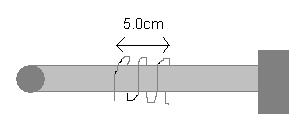# Positioning Device Based On Thermal Expansion

## Homework Statement

In an experiment, a small radioactive source must be moved at extremely slow speeds. A positioning device based on thermal expansion was devised where the radioactive source is attached to one end of an aluminum rod and a heating coil is wrapped around a selection of the rod as shown in the picture below. The rod is fixed on the other end. If the effectively heated section is 5.0cm, at what constant rate do you need to change the temperature of the rod so that the source moves at a constant speed of 50nm/s?## Homework Equations

ΔL = αL0ΔT (linear thermal expansion)

If a body has length L0 at temperature T0, then its length L at a temperature T = T0 + ΔT is:
L = L0 + ΔL = L0 + αL0ΔT = L0(1 + αΔT)

αAluminum = 23 × 10-6/°C

## The Attempt at a Solution

This is a very difficult question due to the fact that we're not given many variables to substitute into an equation. In addition, I have to manipulate the equation so I can introduce "rate" into the equation (length/time). Lastly, we're not given any temperature values either.
That is why I don't really comprehend where and how to start this question. My attempt at the solution so far was brainstorming and analyzing the question but I don't know how to move on. The slightest help with this question will be of great use.

Thank you.

Staff Emeritus

## Homework Equations

ΔL = αL0ΔT (linear thermal expansion)
Differentiate both sides of this wrt time. Show your working...